Related Articles

# Logical and Cartesian Indexing in Julia

• Last Updated : 22 Apr, 2020

Julia, like most technical computing languages, provides a first-class array implementation with very important functions that make working with N-Dimensional arrays quite easy. One of the latest features, the dot(.) based broadcasting makes most of the “repetitive” array operations, a one-lined code.

### Array Indexing

Indexing into arrays in Julia is similar to it’s counterparts.
Syntax:

x = arr[index_1, index_2, …, index_n]

where each index_k may be a scalar integer, an array of integers, or an object that represents an array of scalar indices.

Array Indexing in Julia is of two types:

• Cartesian Indexing
• Logical Indexing

#### Cartesian Indexing

Cartesian Coordinates give the location of a point in 1D, 2D or 3D plane. Cartesian Indices have similar behavior. They give the value of element stored in a 1D, 2D, 3D or n-D array.
For scalar indices, CartesianIndex{N}s, behave like an N-tuple of integers spanning multiple dimensions while array of scalar indices include Arrays of CartesianIndex{N}
Syntax:

```CartesianIndex(i, j, k...)   -> I
CartesianIndex((i, j, k...)) -> I
```

The above syntax creates a multidimensional index I, which can be used for indexing a multidimensional array arr. We can say that, arr[I] is equivalent to arr[i, j, k…]. It is allowed to mix integer and CartesianIndex indices.
Example :

```arr[Ipre, i, Ipost]
(where Ipre and Ipost are CartesianIndex indices and i is an Int)
can be a useful expression when writing algorithms that work along a single dimension
of an array of arbitrary dimensionality.```

#### Scalar CartesianIndexing

CartesianIndexing for 1D arrays:

 `# Create a 1D array of size 5``arr ``=` `reshape(Vector(``1``:``2``:``10``), (``5``))`` ` `# Select index number 1``arr[CartesianIndex(``1``)]`` ` `# Select index number 3``arr[CartesianIndex(``3``)]`

Output: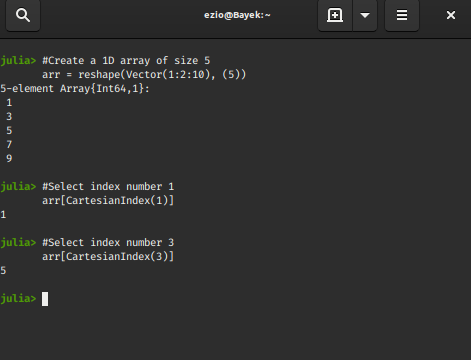CartesianIndexing for a 2D array:

 `# Create a 2D array of size 3x2``arr ``=` `reshape(Vector(``1``:``2``:``12``), (``3``, ``2``))`` ` `# Select cartesian index 2, 2 ``arr[CartesianIndex(``2``, ``2``)]`` ` `# Select index number 3, 1``arr[CartesianIndex(``3``, ``1``)]`

Output: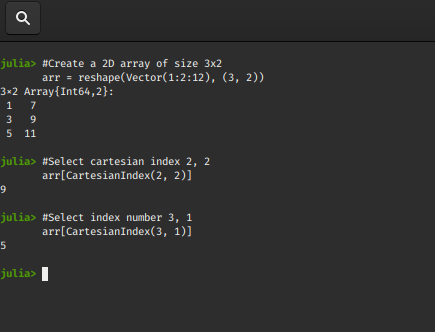CartesianIndexing for 3D Array:

 `# Create a 3D array of size 2x1x2``arr ``=` `reshape(Vector(``1``:``2``:``8``), (``2``, ``1``, ``2``))`` ` `# Select cartesian index 1, 1, 1``arr[CartesianIndex(``1``, ``1``, ``1``)]`` ` `# Select index number 1, 1, 2``arr[CartesianIndex(``1``, ``1``, ``2``)]`

Output: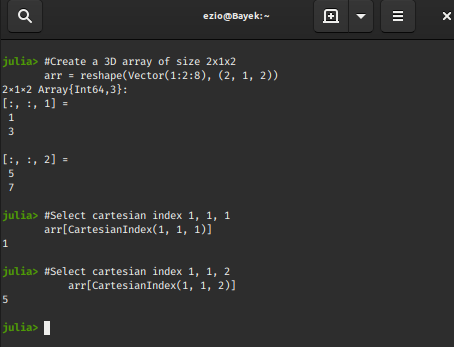CartesianIndexing for nD array:

 `# Create a 5D array of size 2x2x1x2x2``arr ``=` `reshape(Vector(``1``:``2``:``32``), (``2``, ``2``, ``1``, ``2``, ``2``))`` ` `# Select cartesian index 1, 2, 1, 2, 2``arr[CartesianIndex(``2``, ``2``, ``1``, ``2``, ``2``)]`

Output: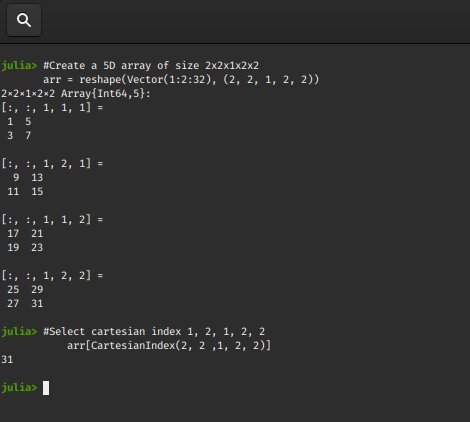From the above examples, it becomes pretty clear that CartesianIndex simply gathers multiple integers together into one object that represents a single multidimensional index.
Having discussed scalar representations, let’s talk about arrays of CartesianIndex{N}, which represent a collection of scalar indices each spanning N dimensions, such form of indexing is referred to as “pointwise” indexing.

#### Array based CartesianIndexing

Array based CartesianIndexing for 1D Array:

 `# Create a 1D array of size 5``arr ``=` `reshape(Vector(``2``:``6``), ``5``)`` ` `# Select an array of cartesian indices 2, 3, 5``arr[[CartesianIndex(``2``), ``     ``CartesianIndex(``3``), CartesianIndex(``5``)]]`

Output: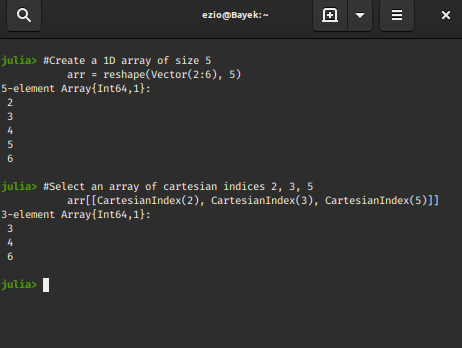Array based CartesianIndexing for 2D Array:

 `# Create a 2D array of size 5x6``arr ``=` `reshape(Vector(``1``:``30``), (``5``, ``6``))`` ` `# Select an array of cartesian indices (5, 2), (3, 6), (1, 4)``arr[[CartesianIndex(``5``, ``2``), ``     ``CartesianIndex(``3``, ``6``), CartesianIndex(``1``, ``4``)]]`

Output: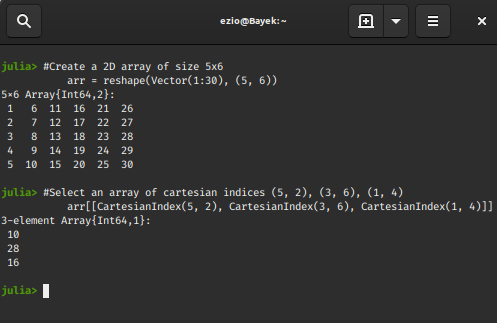Array based CartesianIndexing for 3D array:

 `# Create a 3D array of size 2x3x1``arr ``=` `reshape(Vector(``1``:``12``), (``2``, ``3``, ``2``))`` ` `# Select an array of cartesian indices (1, 2, 1), (2, 2, 2), (1, 3, 1)``arr[[CartesianIndex(``1``, ``2``, ``1``),``     ``CartesianIndex(``1``, ``3``, ``1``), CartesianIndex(``2``, ``2``, ``2``)]]`

Output: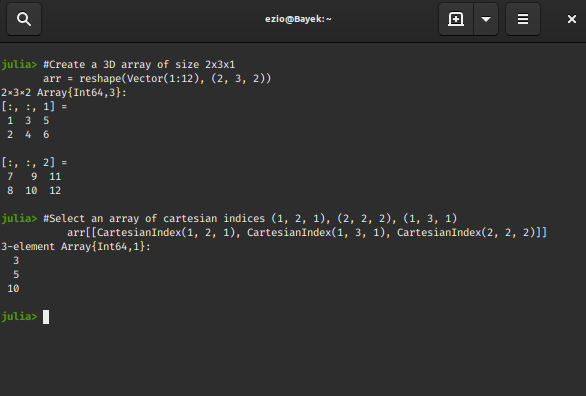Array based CartesianIndexing for nD array:

 `# Create a 5D array of size 2x2x1x2x2``arr ``=` `reshape(Vector(``1``:``2``:``32``), (``2``, ``2``, ``1``, ``2``, ``2``))`` ` `# Select an array of cartesian indices ``# (1, 2, 1, 1, 1), (1, 2, 1, 2, 2), (2, 1, 1, 2, 1)``arr[[CartesianIndex(``1``, ``2``, ``1``, ``1``, ``1``), ``     ``CartesianIndex(``1``, ``2``, ``1``, ``2``, ``2``), ``     ``CartesianIndex(``2``, ``1``, ``1``, ``2``, ``1``)]]`

Output: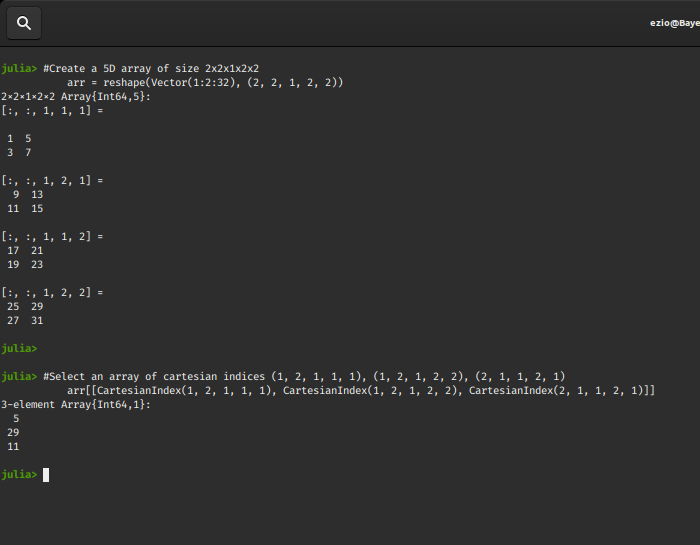This is really useful, in case we want to select specific elements of an array if they are not in order. But what if we have an array of 1000 and we need to extract 100 elements, it’ll be quite tedious!
This is where dot(.) broadcasting comes into play.

 `# Create a 3D array of size 3x3x3``arr ``=` `reshape(Vector(``1``:``27``), (``3``, ``3``, ``3``))`` ` `# Select diagonal elements in all the ``# 3 planes of cartesian coordinates``arr[[CartesianIndex(``1``, ``1``, ``1``), CartesianIndex(``2``, ``2``, ``1``), ``     ``CartesianIndex(``3``, ``3``, ``1``), CartesianIndex(``1``, ``1``, ``2``), ``     ``CartesianIndex(``2``, ``2``, ``2``), CartesianIndex(``3``, ``3``, ``2``),``     ``CartesianIndex(``1``, ``1``, ``3``), CartesianIndex(``2``, ``2``, ``3``), ``     ``CartesianIndex(``3``, ``3``, ``3``)]]`` ` `# Using dot broadcasting and (:)colon, to reduce code``arr[CartesianIndex.(axes(arr, ``1``), axes(arr, ``2``), :)]`

Output: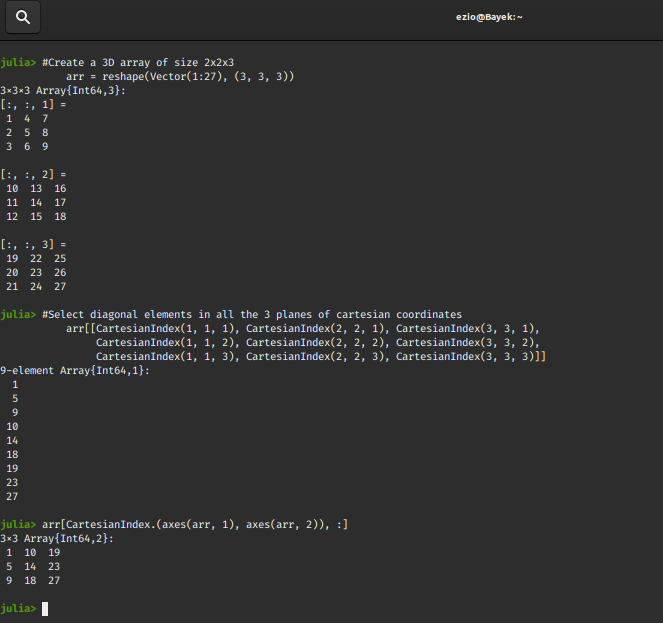How efficiently we have been able to reduce our code in the third step!!

What should we do with Cartesian Indexing ?
We observe, CartesianIndices bear close resemblence to Cartesian coordinates and function in a similar way.
Example:

```We have a 3D shape in space, and it is mapped out by storing the coordinates of it's
edges in an array and we might want to mask out a region from the shape. It becomes
easy to deal with by using CartesianIndices, since they resemble the coordinate system.
```

#### Logical Indexing

In computing/electronics, the basis is a logic that is deterministic in nature. It is:

```true, false
one, zero
on, off
```

It is basically a selection of elements at the indices where the values of our logical indexing array are true.
A logical indexing array is called a “mask” since it masks out the values that are false. A mask is of type bool(boolean).

Relation with CartesianIndexing:

```Indexing by a N-dimensional boolean array is equivalent to indexing by the vector
of CartesianIndex{N}s where its values are true.
```

Example : Implementation of a logical mask

 `# Create a 2D array of size 5x5``arr ``=` `reshape(Vector(``1``:``25``), (``5``, ``5``))`` ` `# Apply a logical mask to the array``arr[[true, false, true, false, true], :]`

Output: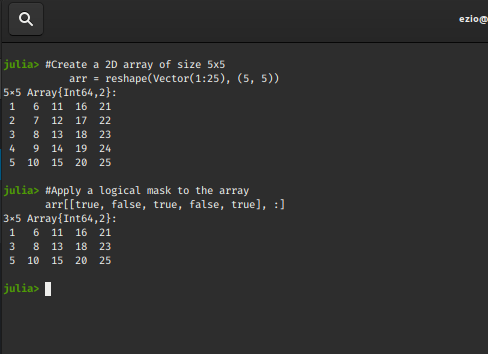So we see how only the rows whose index matches with the index of trues in our mask[true, false, true, false, true] are selected.

Any condition that yields a boolean value can be used as a mask.
Example for 1D array:

 `# Create a 1D array of size 10``arr ``=` `reshape(Vector(``4``:``2``:``22``), ``10``)`` ` `# Create a power of 2 logic mask ``# and map it with the size of arr``mask ``=` `map``(ispow2, arr)`` ` `# Apply it to the array``arr[mask]`

Output: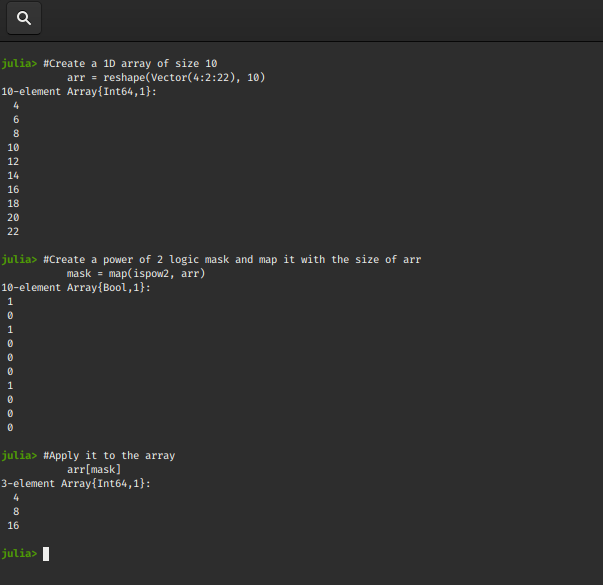Example for 2D array:

 `# Create a 2D array of size 10x10``arr ``=` `reshape(Vector(``1``:``100``), (``10``, ``10``))`` ` `# Create a prime number logic mask and ``# map it with the size of arr ``# Julia library for working with prime numbers ``using Primes ``mask ``=` `map``(isprime, arr)`` ` `# Apply it to the array``arr[mask]`

Output: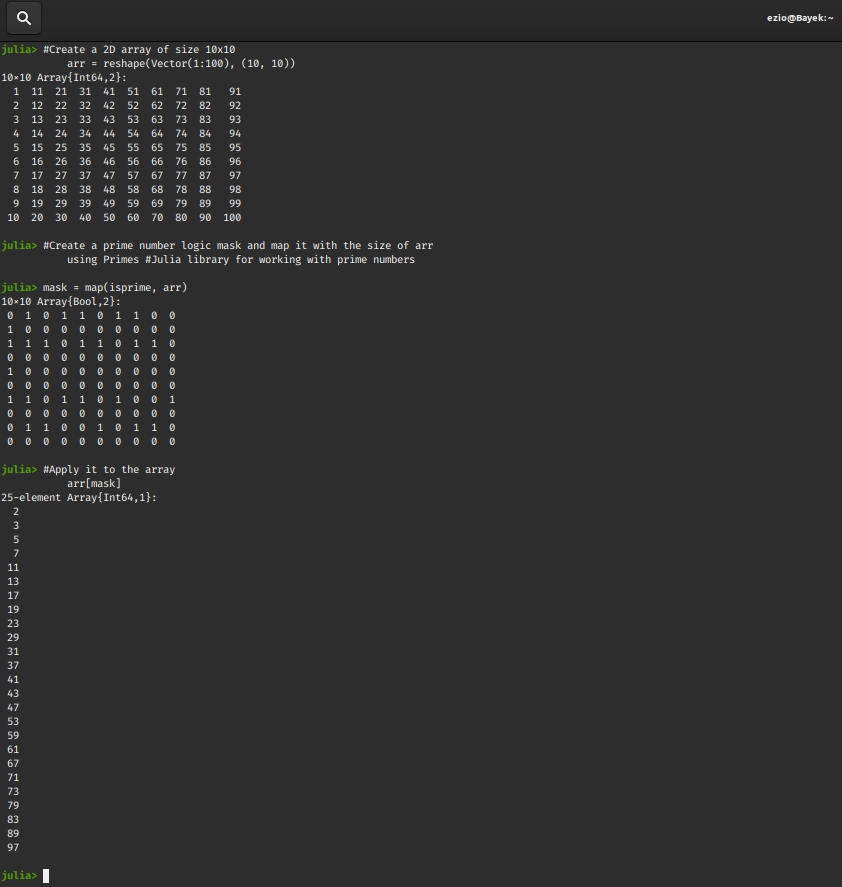Example for 3D array:

 `# Create a 3D array of size 3x2x3``arr ``=` `reshape(Vector(``2``:``19``), (``3``, ``2``, ``3``))`` ` `# Create a prime number logic mask ``# and map it with the size of arr``# Julia library for working with prime numbers ``using Primes``mask ``=` `map``(isprime, arr)`` ` `# Apply it to the array``arr[mask]`

Output: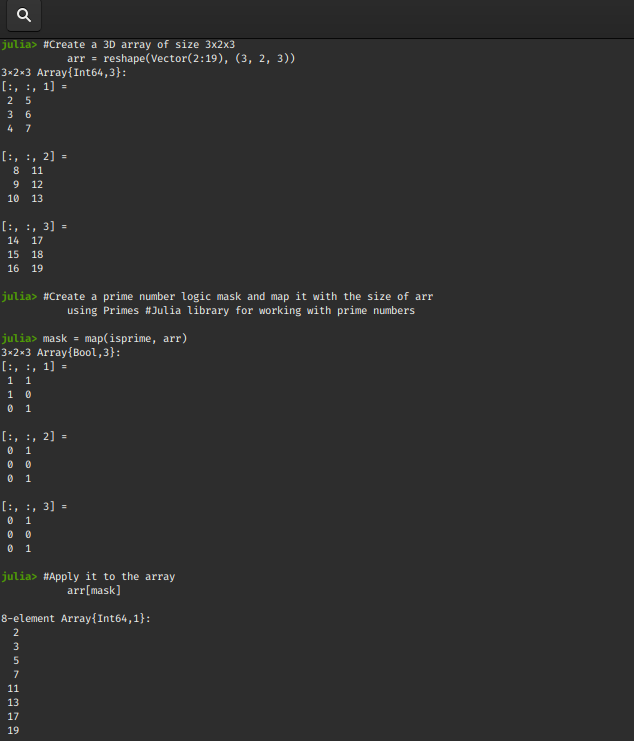One of the most used cases of logical indexing is when we want to filter elements out of an array on the basis of multiple conditions.
Example:

 `# Create a 3D array of size 3x2x3``arr = reshape(Vector(2:19), (3, 2, 3))`` ` `# Create a function that checks for ``# both prime number or power of two``# Julia library for working with prime numbers ``using Primes   ``function prime_and_2pow(arr)``    ``if isprime(arr) || ispow2(arr)``        ``return true``    ``else ``        ``return false``    ``end``end`` ` `# Create a logic mask and map it``# with the size of arr``mask = map(prime_and_2pow, arr)``     ` `# Apply it to the array``arr[mask]`

Output: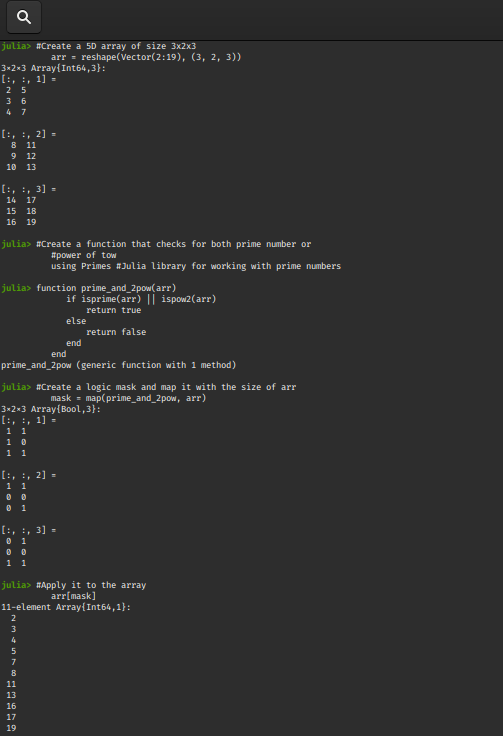My Personal Notes arrow_drop_up